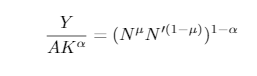# Problem to find steady state when I assume Y=1

Hello, I am trying to do a DSGE model in Matlab, I have all the steady state equations on paper and pencil but I fail to find values for all variables. Here is my mod file: new.mod (6.6 KB)

Indeed, since I have constant return to scale,I have tried to assume Y=1 to simplify the calculations. But it leads me to a problem:

My market clearing equation is okay: all sums to 1 at steady state.However the production function does not equal to 1. It’s because I have used other equations to determine N, N prime and wages. I used these equations to determine N, N ‘, w and w’:I have tried to use the production function, make Y=1, and since I know K I can express N in function of N '.Where Y=A=1 and K is known

But then I fail at finding on paper and pencil a value for N, N prime and w and w’

Do you have an idea how can I assume Y=1 and still have the market clearing and the production function equal to 1 ? Do you recommand me another way to solve my steady states ?

I don’t think you can assume steady-state values for endogenous variables like Y_t. They are endogenous variables and they need to be determined by the parameters in the model. The steady-state values of all endogenous variables should be a function of the structural parameters in the model. So, for example, Y = f (\alpha, \mu,...) for your model and f is unique and can be found. You can’t just assume values for Y and at the same time assume values for the parameters (\alpha, \mu,...) when you do not know what f is. If you pick any values for Y and any values for (\alpha, \mu,...), the equality will not hold. It is nearly impossible to randomly pick Y = 1, and some values for the parameters in the model (\alpha, \mu,...) such that Y = f (\alpha, \mu,...) if you don’t know what f is.

For sure, f exists if your model has a steady-state, and you can find it. After you find f, you can assume values for (\alpha, \mu,...) and then find Y. This is called calibration.

Also since Y = f (\alpha, \mu,...) is just a mapping, you can also assume Y=1 and then use that to calculate values for the parameters (\alpha, \mu,...). This is an alternative way of calibration. For example, if Y = 1 in the data, i.e., the long-run average of real GDP per capita = 1 in your data, you can assume Y = 1 in the model and then calculate the parameters (\alpha, \mu,...).

But in any case, you must first find the function f that maps Y to the parameters (\alpha, \mu,...). If you just assume arbitrary values on both sides of Y = f (\alpha, \mu,...) just to simplify things, it will not work since the model has a unique steady-state solution defined by a unique f which you must find.

For exogenous variables like A_t, of course, you can assume values for it because they are not determined by the parameters in the model. In other words, they are exogenous, so yeah, you can assume A_t =1

Thank you for your answer. However I disagree with you. Let me explain.

First jpfeifer told me in a previous post that I can assume Y=1 if and only if I have constant return to scale technology and a productivity parameter A to scale the economy:

Secondly, I tried to do so and I’ve seen that Eric Sims do so in his replication of Iacoviello (2005) paper where he assumes productivity parameter A to scale the economy like in these pics (page 21 of this PDF Iacoviello 2005 + détaillé.pdf (456.2 KB) ) :

This is what did, I assumed A to be constant to have Y=1, and now my problem is solved I think.

1 Like

I don’t really see how you could set both Y=1 and N=1 without additionally scaling N via a second parameter that then becomes an endogenous object in steady state.

Thank you for your answer. I think I expressed myself in a wrong way. I have set Y=1 and it enabled me to find N that is different from 1. But to assume Y=1 I needed to set the value of the productivity parameter A accordingly, as Eric Sims did in the screenshot I uploaded above.

Ok. I only saw that in the posted mod-file A=1.

Of course, I never said you can’t assume Y = 1. I only said given Y = f(\alpha, \mu, ...), you cannot assume any values you want for both sides of the equation. And putting ‘...’ means there are more parameters in there besides \alpha and \mu, like A. If you fix Y=1 and you know what the function f is, you can calculate, for example, A that will be consistent with Y=1 given the other parameters in the model. This is what Sims did. He calibrated A to be consistent with Y = 1.

But in your question, you fixed A = Y= 1. If you want to fix A=1, then you should calibrate some other parameter, say \alpha = g(Y=1,A=1,\mu...) to be consistent with Y = 1.

You cannot assume any values for all parameters that determine Y including A and expect to find the steady-state.

As you said, you need to set A accordingly. But you can also fix A=1 as you did in your question, and set \alpha or \mu or some other parameter accordingly. It is not always A.